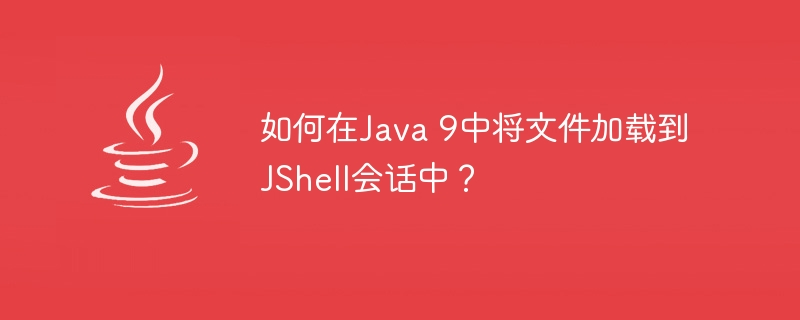# 如何在Java 9中将文件加载到JShell会话中？JShell 是一个新的命令行交互式 REPL (Read-Evaluate-Print-Loop) 工具Java 9中引入，用于评估用 Java 编写的声明、语句和表达式。该工具还允许我们执行Java 代码片段并立即获得结果。

```String s1 = "TutorialsPoint";
String s2 = "Tutorix";
String s3 = s1 + s2;

int sum(int a, int b) {
return a + b;
}

int divide(int a, int b) {
return a / b;
}```

```C:\Users\User>jshell
| Welcome to JShell -- Version 9.0.4
| For an introduction type: /help intro

jshell> /open c:\temp\Test.java

jshell> /vars
|    String s1 = "TutorialsPoint"
|    String s2 = "Tutorix"
|    String s3 = "TutorialsPointTutorix"

jshell> /methods
|    int sum(int,int)
|    int divide(int,int)```

" /open "命令已将 "Test.java"文件加载到会话中。" /vars "命令可用于将变量加载到会话中，" /methods "命令可用于将方法加载到会话中。